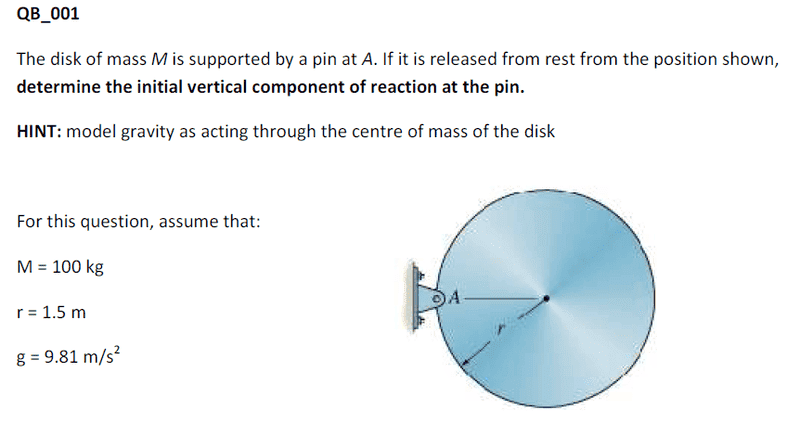# Dynamics: disc released horizontally, with pin fixed to edge (rotation)

Hi guys, im really stumped by this 1st yr Dynamics question, and would appreciate any help on how to approach the question.

## Homework Statement## Homework Equations

Torque equations and Force equations
Moment of inertia for disc rotating around centre: (mr^2)/2
After using parallel axis theorem, the I about the edge is I = (3/2)mr^2

Thank you,
Aralox

Redbelly98
Staff Emeritus
Homework Helper
Welcome to PF.

## Homework Equations

Torque equations and Force equations
Moment of inertia for disc rotating around centre: (mr^2)/2
After using parallel axis theorem, the I about the edge is I = (3/2)mr^2

Thank you,
Aralox
Using the parallel axis theorem is a good start. Can you do the following:

1. Given I, and the torque about the pivot point, can you calculate the angular acceleration of the disk?

2. From the angular acceleration about the pivot, you should be able to figure out the acceleration of the disk's center of mass.

1. Given I, and the torque about the pivot point, can you calculate the angular acceleration of the disk?
I used Net Torque = mgr, as initially the weight is perp. to radius to pivot.
Since net torque = Iα (α is angular acc.),
mgr = Iα.
Since I = (3/2) mr^2, I simplified the previous equation to

α = 2g/3r, which happens to be a constant, so it looks like i have the initial angular acceleration of the disc about the pivot at the edge.

2. From the angular acceleration about the pivot, you should be able to figure out the acceleration of the disk's center of mass.
To find this, i used the eqation a = αr,
so acceleration of disc's center: a = (2g/3r) * r = (2/3)g

How would i find the vertical reaction of the pin from this though?
Just brainstorming, could i do something where i took the disc to be not moving and the world rotating around it, meaning i would have the edge moving at a = (2/3)g, which i could get some kind of reaction force from?
This interpretation/application step is what im having the most trouble with.

Thanks,
Aralox

PS: Just a test to figure out this 'latex reference' thing
$\sum Torque$

Redbelly98
Staff Emeritus
Homework Helper
Looks good so far.
.
.
...so acceleration of disc's center: a = (2g/3r) * r = (2/3)g

How would i find the vertical reaction of the pin from this though?
Just brainstorming, could i do something where i took the disc to be not moving and the world rotating around it, meaning i would have the edge moving at a = (2/3)g, which i could get some kind of reaction force from?
This interpretation/application step is what im having the most trouble with.
Well, now that you know the acceleration of the disk's center of mass, you pretty much know what the net force (vector sum of all forces) acting on the disk must be...

$\sum F$ = N - mg = ma,
which leads to N = (5/3)mg

would N be the 'vertical component of reaction at the pin'? Isnt N the force (reaction force of some sort) vector originating at the centre of the disc, since im taking net force at the centre?

Just a little bump :)

Redbelly98
Staff Emeritus
Homework Helper
Sorry, somehow I missed your earlier post.

$\sum F$ = N - mg = ma,
which leads to N = (5/3)mg
Well, a is downward, right? And you're taking the downward direction to be negative.

would N be the 'vertical component of reaction at the pin'?
Yes.
Isnt N the force (reaction force of some sort) vector originating at the centre of the disc, since im taking net force at the centre?
No, N is acting at the pin. You're taking the net force acting on the disk, meaning the vector sum of all forces acting on the disk, whether they act at the center or not.

Ohhhhh now i understand! I've always had this idea in my head that when taking the net force they must all act at the same point, but now i think about it, that cant be the case.

Thank you for all the help Redbelly98! :)